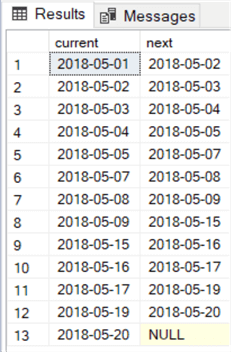# SQL Server Window Functions Gaps and Islands Problem

##### Overview

In this last part of the tutorial, we’ll look into one final use case that can be solved with window functions: gaps and islands.

##### Explanation

The gaps and islands problem is about finding holes in sequences (the gaps) or about finding ranges of consecutive values (the islands). Typical examples are: how long and how frequent has someone logged into a website (island problem)? What were the sick days of a person and did the number of sick days over a period of time surpassed some value so that it is now extended sick leave (also an island problem)? How often did the server go offline (gaps problem)? When did the student not attend the classes (gaps problem)?

Both problems are related to each other, as finding gaps is the inverse of finding islands. Let’s analyze the problem where a server sometimes goes offline.

## Gaps

First we’re going to find out on which days the server went offline. Maybe there’s a correlation with scheduled maintenance? The following T-SQL query generates some sample data:

```CREATE TABLE dbo.[Server]
(ID         INT IDENTITY(1,1) NOT NULL
,ServerName VARCHAR(50) NOT NULL
,DayOnline  DATE NOT NULL);

INSERT INTO dbo.[Server]
(
[ServerName]
,DayOnline
)
VALUES
('MyServer','2018-05-01')
,('MyServer','2018-05-02')
,('MyServer','2018-05-03')
,('MyServer','2018-05-04')
,('MyServer','2018-05-05')
,('MyServer','2018-05-07')
,('MyServer','2018-05-08')
,('MyServer','2018-05-09')
,('MyServer','2018-05-15')
,('MyServer','2018-05-16')
,('MyServer','2018-05-17')
,('MyServer','2018-05-19')
,('MyServer','2018-05-20');
```

We want to find out that on the 6th and 18th of May 2018 the server was offline, as well as the whole range of 10 till 14th of May.

For each row, find the current date and the date from the next row. This can be accomplished by using LEAD (part 7):

```SELECT
[current] = [DayOnline]
,[next]    = LEAD([DayOnline]) OVER (ORDER BY [DayOnline])
FROM [dbo].[Server];
```

The result:We can now identify all gaps by finding all rows where the difference between the current and the next date is bigger than one.

```SELECT
FROM
(
SELECT
[current] = [DayOnline]
,[next]    = LEAD([DayOnline]) OVER (ORDER BY [DayOnline])
FROM [dbo].[Server]
) tmp
WHERE DATEDIFF(DAY,[current],[next]) > 1;
```

We have now isolated the gaps:## Islands

Let’s reverse the problem and try to find the islands: the periods in time where the server was online. You can use this to calculate uptime for example. First we’re going to add a dense rank:

```SELECT
[DayOnline]
,DRank = DENSE_RANK() OVER (PARTITION BY [ServerName] ORDER BY [DayOnline])
FROM [dbo].[Server];
```Now we’re going to subtract this rank from the date. For every consecutive value in a range, the same date will be returned as a result. This will act as some sort of grouping.

```SELECT
[DayOnline]
,-1 * DENSE_RANK() OVER (PARTITION BY [ServerName]
ORDER BY [DayOnline])
,[DayOnline])
FROM [dbo].[Server];
```Now we can use that grouping to find the minimum date (the start of the range) and the maximum date (the end of the range).

```SELECT
StartDate  = MIN([tmp].[DayOnline])
,EndDate    = MAX([tmp].[DayOnline])
FROM
(
SELECT
[DayOnline]
,-1 * DENSE_RANK() OVER (PARTITION BY [ServerName]
ORDER BY [DayOnline])
,[DayOnline])
FROM [dbo].[Server]
) tmp
GROUP BY [tmp].[Group];
```

And we have found our islands: